# para2fan

Convert parallel-beam projections to fan-beam

## Syntax

F = para2fan(P,D)
F = para2fan(P,D,Name,Value)
[F,fanSensorPos,fanRotAngles] = para2fan(___)

## Description

example

F = para2fan(P,D) converts the parallel-beam data P to the fan-beam data F. Each column of P contains the parallel-beam sensor samples at one rotation angle. D is the distance from the fan-beam vertex to the center of rotation.The parallel-beam sensors are assumed to have a one-pixel spacing. The parallel-beam rotation angles are spaced equally to cover [0, 180] degrees. The calculated fan-beam rotation angles have the same spacing as the parallel-beam rotation angles, and cover [0,360) degrees. The calculated fan-beam angles are equally spaced with the spacing set to the smallest angle implied by the sensor spacing.
F = para2fan(P,D,Name,Value) uses name-value arguments to control aspects of the data conversion.
[F,fanSensorPos,fanRotAngles] = para2fan(___) returns the fan-beam sensor locations in fanSensorPos and rotation angles in fanRotAngles.

## Examples

collapse all

Generate parallel-beam projections

ph = phantom(128); theta = 0:180; [P,xp] = radon(ph,theta); imshow(P,[],'XData',theta,'YData',xp,'InitialMagnification','fit') axis normal title('Parallel-Beam Projections') xlabel('\theta (degrees)') ylabel('x''') colormap(gca,hot), colorbarConvert to fan-beam projections

[F,Fpos,Fangles] = para2fan(P,100); figure imshow(F,[],'XData',Fangles,'YData',Fpos,'InitialMagnification','fit') axis normal title('Fan-Beam Projections') xlabel('\theta (degrees)') ylabel('Sensor Locations (degrees)') colormap(gca,hot), colorbar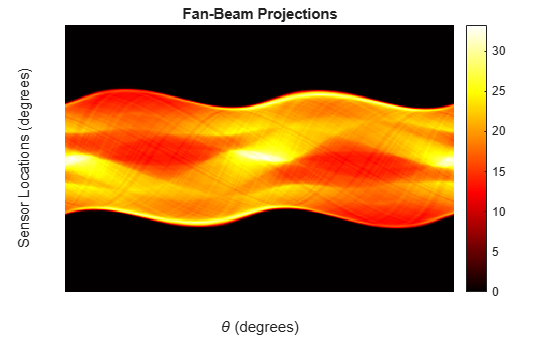## Input Arguments

collapse all

Parallel-beam projection data, specified as a numeric matrix. Each column of P contains the parallel-beam data at one rotation angle. The number of columns indicates the number of parallel-beam rotation angles and the number of rows indicates the number of parallel-beam sensors.

Data Types: double | single

Distance in pixels from the fan beam vertex to the center of rotation, specified as a positive number. para2fan assumes that the center of rotation is the center point of the projections, which is defined as ceil(size(F,1)/2). The value of D must be greater than or equal to ParallelSensorSpacing*(size(P,1)-1)/2. The figure illustrates D in relation to the fan-beam vertex for one fan-beam projection.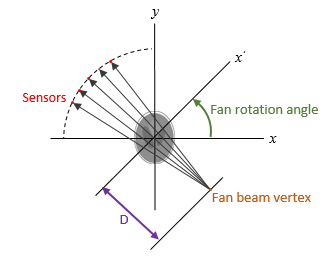Data Types: double | single

### Name-Value Arguments

Specify optional pairs of arguments as Name1=Value1,...,NameN=ValueN, where Name is the argument name and Value is the corresponding value. Name-value arguments must appear after other arguments, but the order of the pairs does not matter.

Example: F = para2fan(P,D,FanRotationIncrement=5) specifies a fan rotation increment of 5 degrees.

Before R2021a, use commas to separate each name and value, and enclose Name in quotes.

Example: F = para2fan(P,D,"FanRotationIncrement",5) specifies a fan rotation increment of 5 degrees.

Range of fan-beam rotation, specified as "cycle" or "minimal".

• "cycle" — Rotate through the full range [0, 360) degrees.

• "minimal" — Rotate through the minimum range necessary to represent the object.

Fan-beam rotation angle increment in degrees, specified as a positive scalar.

• If FanCoverage is "cycle", then 360/FanRotationIncrement must be an integer.

• If you do not specify FanRotationIncrement, then the default value is equal to the parallel-beam rotation angle.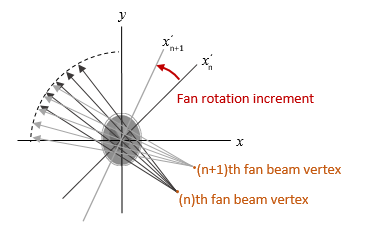Data Types: double

Fan-beam sensor positioning, specified as "arc" or "line".

Value

Meaning

Diagram

"arc"

Sensors are spaced at equal angles along a circular arc. The center of the arc is the fan-beam vertex.

FanSensorSpacing defines the angular spacing in degrees.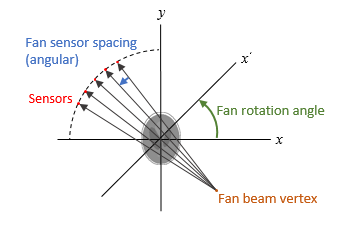"line"

Sensors are spaced at equal distances along a line that is parallel to the x' axis. The closest sensor is distance D from the center of rotation.

FanSensorSpacing defines the distance between fan-beams on the x' axis, in pixels.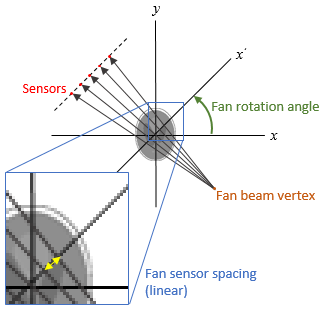Fan-beam sensor spacing, specified as a positive scalar.

• If FanSensorGeometry is "arc", then FanSensorSpacing defines the angular spacing in degrees.

• If FanSensorGeometry is "line", then FanSensorSpacing defines the linear distance between fan-beams, in pixels. Linear spacing is measured on the x' axis.

If you do not specify FanSensorGeometry, then the default value of FanSensorSpacing is the smallest value implied by ParallelSensorSpacing such that:

• If FanSensorGeometry is "arc", then FanSensorSpacing is 180/pi*asin(ParallelSensorSpacing/D)

• If FanSensorGeometry is "line", then FanSensorSpacing is D*asin(ParallelSensorSpacing/D)

Data Types: double

Type of interpolation used between the parallel-beam and fan-beam data, specified as one of these values.

"nearest" — Nearest-neighbor

"linear" — Linear (the default)

"spline" — Piecewise cubic spline

"pchip" — Piecewise cubic Hermite (PCHIP)

Range of parallel-beam rotation, specified as "halfcycle" or "cycle".

• "cycle" — Parallel data covers the full range of [0, 360) degrees.

• "halfcycle" — Parallel data covers [0, 180) degrees.

Parallel-beam sensor spacing in pixels, specified as a positive scalar.

Data Types: double

## Output Arguments

collapse all

Fan-beam projection data, returned as a numeric matrix. Each column of F contains the fan-beam sensor samples at one rotation angle.

Parallel-beam projection data, returned as a numeric matrix. Each column of F contains the fan-beam data at one rotation angle. The number of columns indicates the total number of fan-beam rotation angles and is equal to the length of fanRotAngles. The number of rows indicates the total number of parallel-beam sensors and is equal to the length of fanSensorPos.

Data Types: double

Fan-beam sensor locations, returned as a numeric column vector.

• If FanSensorGeometry is "arc" (the default), then fanSensorPos contains the fan-beam sensor measurement angles.

• If FanSensorGeometry is "line", then fanSensorPos contains the fan-beam sensor positions along the line of sensors.

Data Types: double

Fan-beam rotation angles, returned as a numeric row vector.

Data Types: double

## Version History

Introduced before R2006a